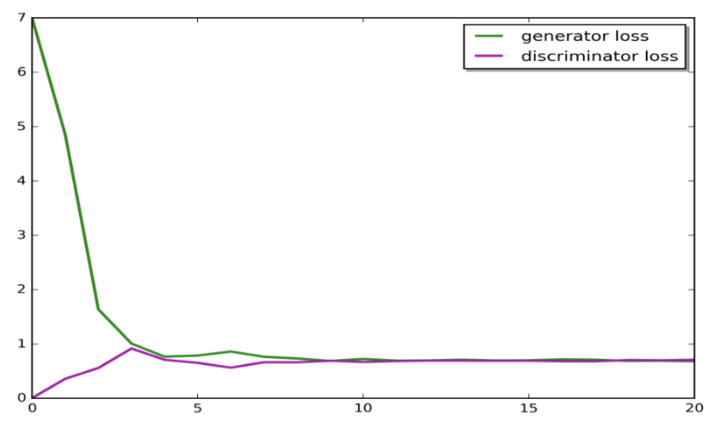#### What is GAN:

GAN are Generative Adversarial networks. GANs are neural networks that generate synthetic data given certain input data. The main goal is unsupervised sampling from complex high dimensional distribution. And this is done by taking samples from random noise and learn the transformation to input distribution.

These are a type of generative model, because they learn to copy the data distribution of the data you give it, and therefore can generate novel images that look alike. The reason why a GAN is named “adversarial”, is because it involves two competing networks (adversaries), that try to outwit each other.

#### Turing Learning:

Turing Learning is the generalization of the procedure underlying GAN. The word ‘Turing’ comes from the similarities to the Turing test, in which a computer tries to fool the system into thinking that it is a human. As we will see, this is analogous to the goals of the generator in a GAN, which tries to fool its ‘adversary’, the discriminator. The need for having a generalization of GANs stems from the fact that Turing learning can be performed with any form of generator or discriminator, not necessarily a neural network.

The main reason that using neural networks is commonplace within Turing learning is the fact that a neural network is a universal function approximator. That is, we can use a neural network (assuming it has enough capacity, i.e., many nodes) to ‘learn’ a non-linear mapping between the input and the output. Also, the replacing neural networks with SVM or other
Types leads to increase in the bias of the model. GANs have no explicit density function defined. They are direct implementations of the implicit density.

#### Taxonomy:

Generative Models are used to generate synthetic data from given input data. It is segregated into two types viz – Explicit, Implicit. In Explicit density generative models, the density distribution of the input data is defined and the likelihood of this distribution is maximized. Some of the models are pixelRNN/CNN, variational Auto encoders. In Implicit density Generative Models, the density function whose likelihood is to be maximized is not defined but is learnt by the model itself. GANs belong to this category of Implicit density generative models.

#### Generator and Discriminator:

Generative Adversarial Networks consists of two models; generator and discriminator

#### The Discriminative Model:

The discriminative model operates like a normal binary classifier that’s able to classify images into different categories. It determines whether an image is real and from a given dataset or is artificially generated. The Discriminator does the job of a police by trying to distinguish between real and fake images(counterfeits).

#### The Generative Model:

The discriminative model tries to predict certain classes given certain features. The generative model tries to predict features given classes. This involves determining the probability of a feature given a class. It acts as a counterfeiter by trying to fool the discriminator by generating real-looking images(counterfeits).

In GANs, the generative model is pitted against an adversary: a discriminative model that learns to determine whether a sample is from the model distribution or the data distribution. The generative model can be thought of as analogous to a team of counterfeiters, trying to produce fake currency and use it without detection, while the discriminative model is analogous to the police, trying to detect the counterfeit currency. Competition in this game drives both teams to improve their methods until the counterfeits are undistinguished from the genuine articles.

To briefly summarize how this works, a random sample is taken from some prior distribution, which is fed into the generator to make some fake image. This fake image, along with the real data, is fed into the discriminator network, which then decides which data comes from the real data set, and which comes from the fake data generated from the prior distribution.

#### MINIMAX Game:

Zero-sum game: Players compete for a fixed and limited pool of resources. Players compete for resources, claiming them and each player’s total number of resources can change, but the total number of resources remain constant.

In zero-sum games, each player can try to set things up so that the other player’s best move is of as little advantage as possible. This is called a minimax, or minmax, technique.

Our goal in training the GAN is to produce two networks that are each as good as they can be. In other words, we don’t end up with a “winner.”

Instead, both networks have reached their peak ability given the other network’s abilities to thwart it. Game theorists call this state a Nash equilibrium, where each network is at its best configuration with respect to the other.Figure: Loss function vs. number of epochs for discriminator and generator networks — flat line is Nash equilibrium.

#### Network Training:

The goal of the discriminator is to maximize the overall value function whereas that of the Generator (G) is minimize the discriminator’s value function/The training process is basically alternating between Gradient ascent on D and Gradient descent on G.

The Discriminator tries to make D(X) near to 1 (predict real on training examples), D(G(z)) near to 0(predict fake on images generated by the generator)

The Generator tries to fool the discriminator so it always tries to make D(G(z)) to be 1. This scenario is a minimax game between generator and discriminator.

The above figure shows the algorithm for training of the generator and discriminator. Initially we start with training the discriminator for a certain number of steps (k, which is a hyperparameter) later on the generator is updated. Deciding the number of steps for which the discriminator needs to be trained is based on the application and is still an active area of research.